Ex 4.5

Chapter 4 Class 12 Determinants
Serial order wise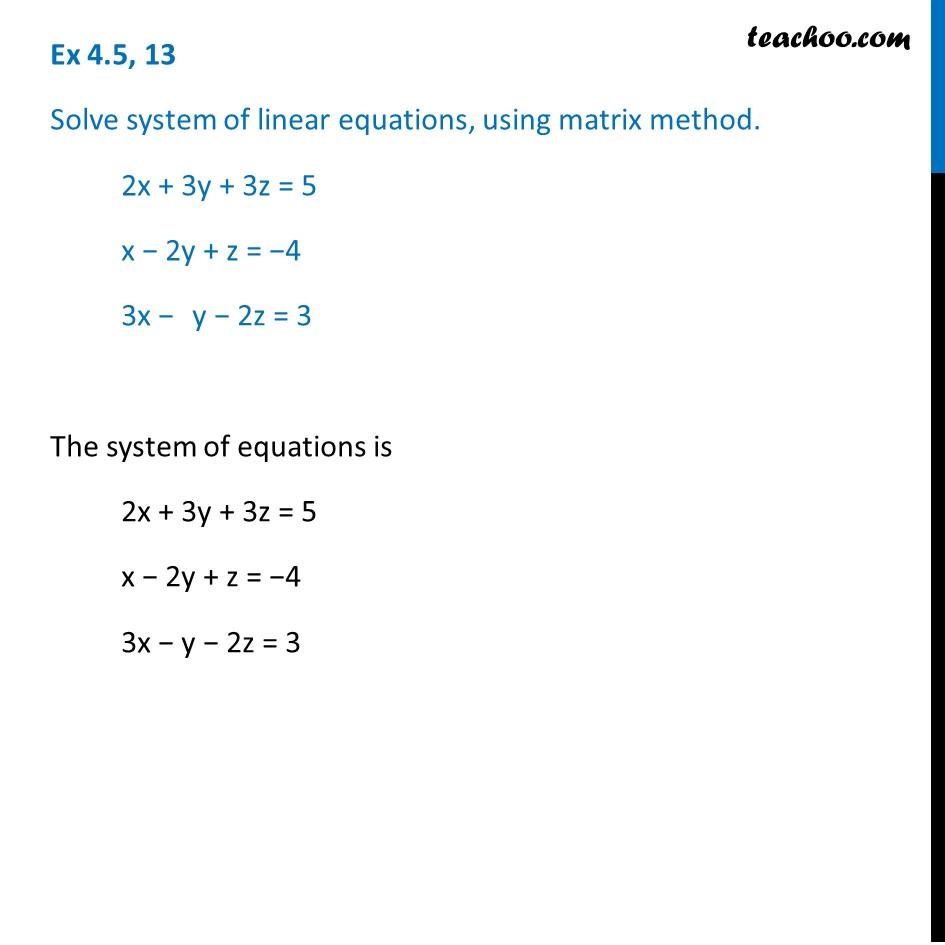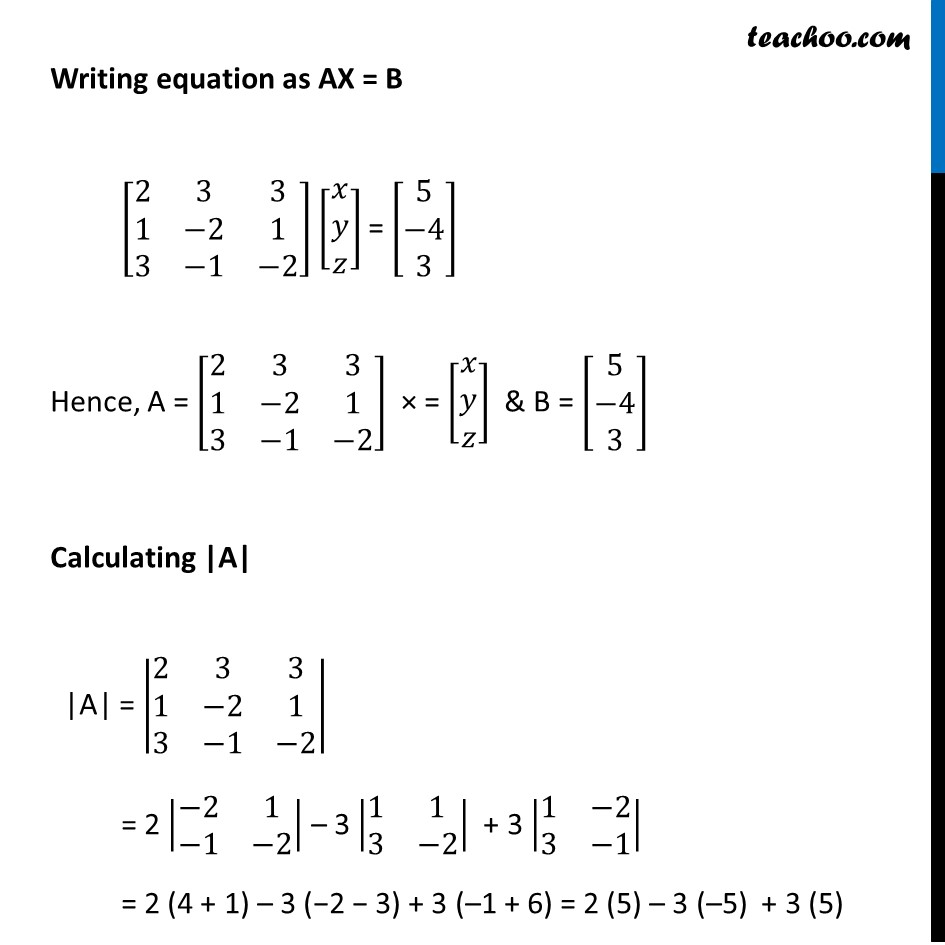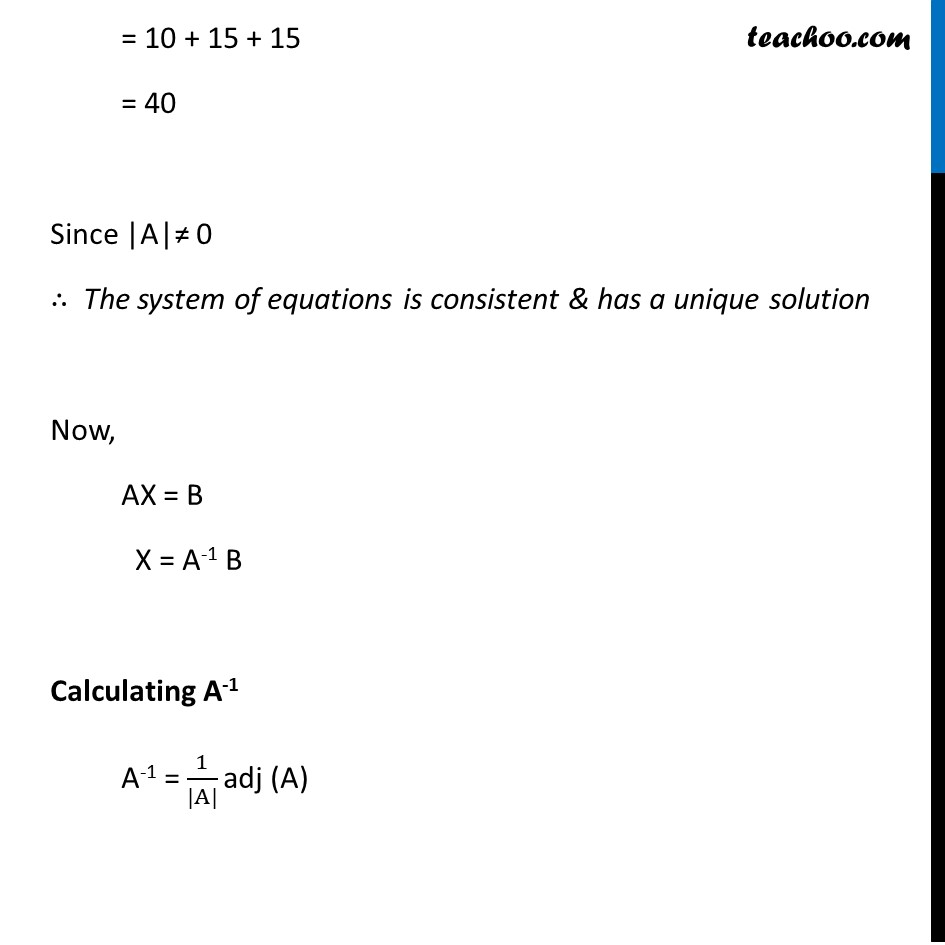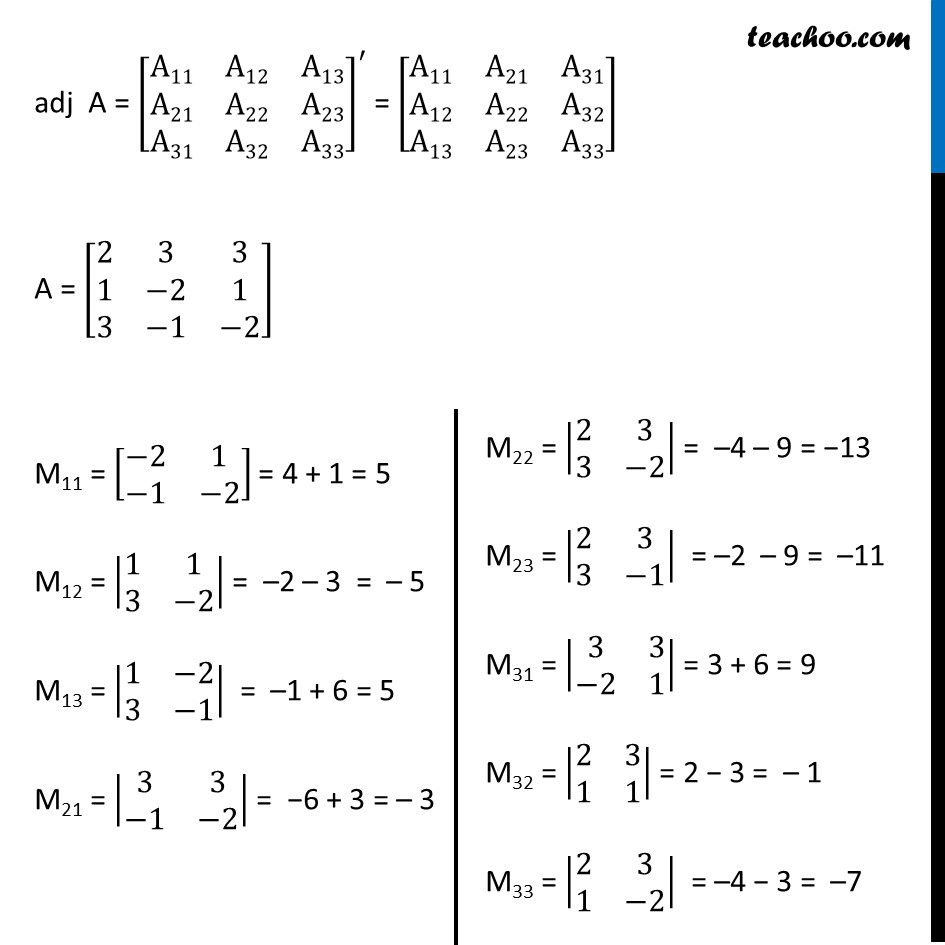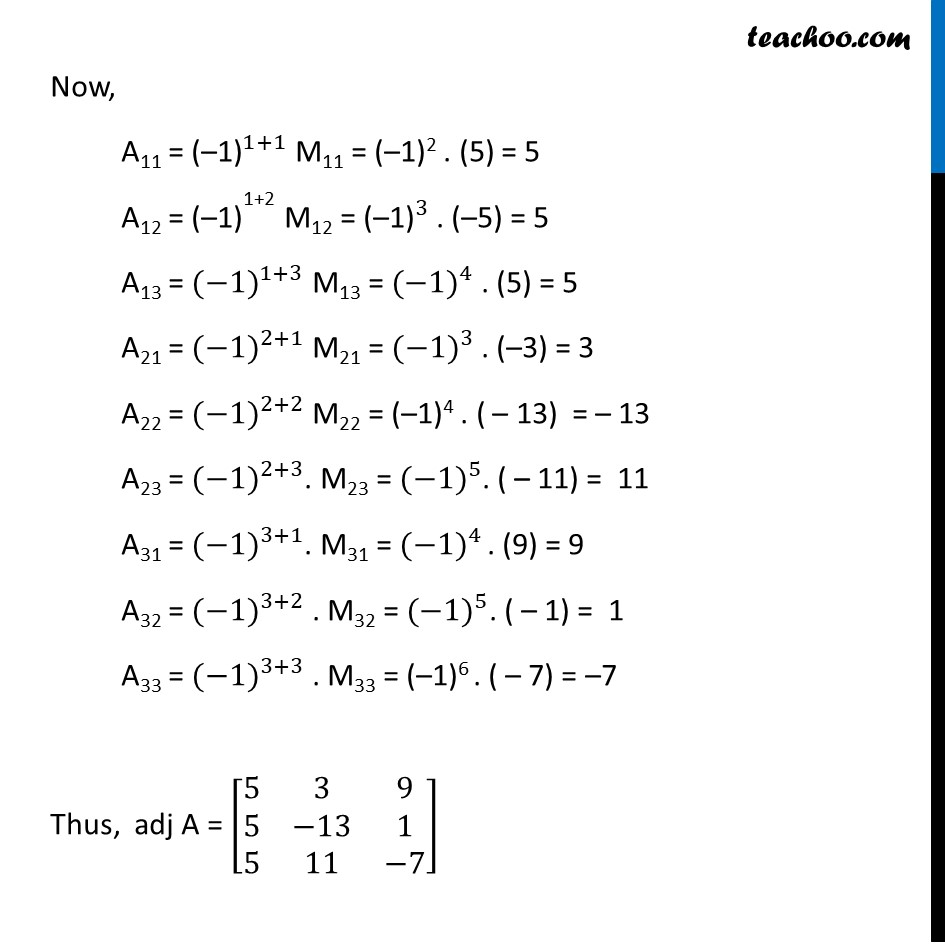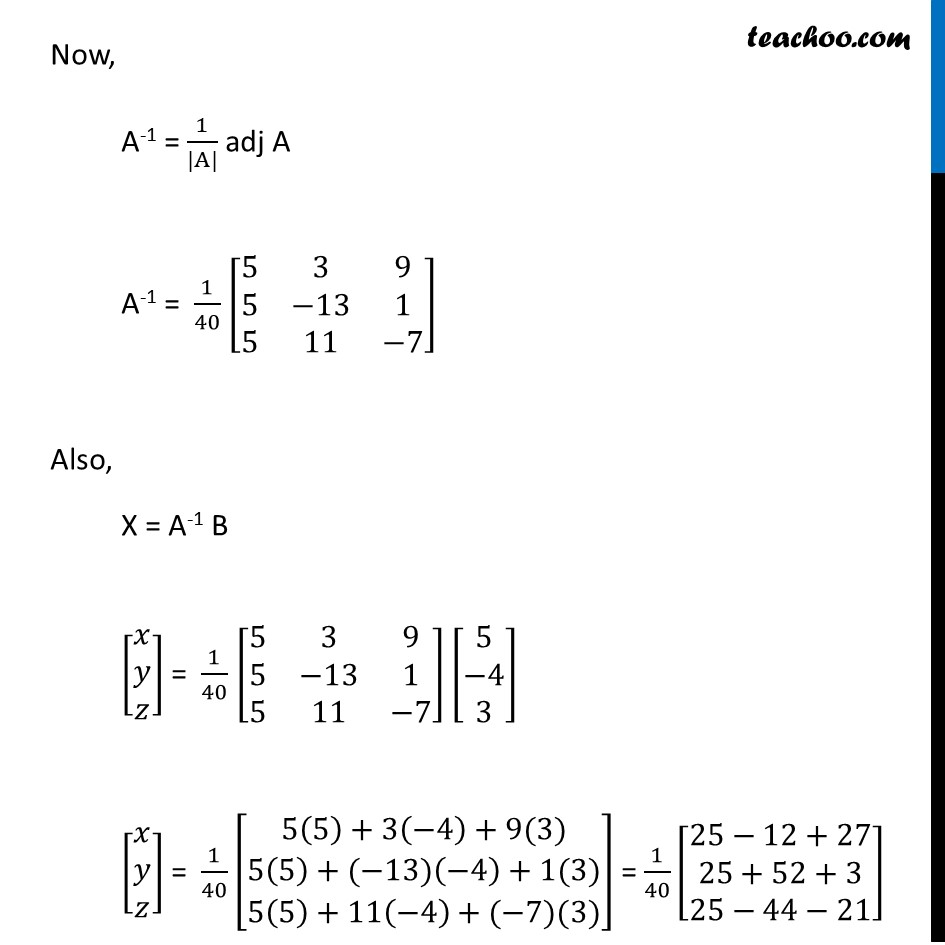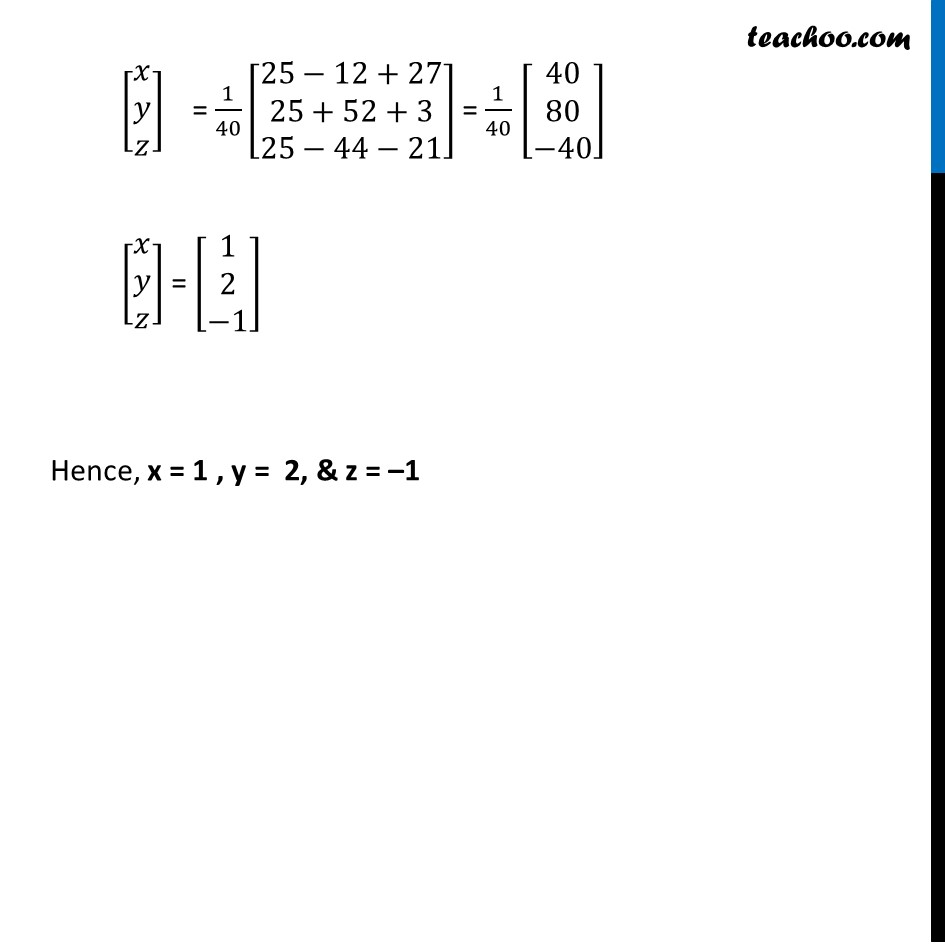Learn in your speed, with individual attention - Teachoo Maths 1-on-1 Class

### Transcript

Ex 4.5, 13 Solve system of linear equations, using matrix method. 2x + 3y + 3z = 5 x − 2y + z = −4 3x − y − 2z = 3 The system of equations is 2x + 3y + 3z = 5 x − 2y + z = −4 3x − y − 2z = 3 Writing equation as AX = B [■8(2&3&3@1&−2&1@3&−1&−2)] [■8(𝑥@𝑦@𝑧)] = [■8(5@−4@3)] Hence, A = [■8(2&3&3@1&−2&1@3&−1&−2)] × = [■8(𝑥@𝑦@𝑧)] & B = [■8(5@−4@3)] Calculating |A| |A| = |■8(2&3&3@1&−2&1@3&−1&−2)| = 2 |■8(−2&1@−1&−2)| – 3 |■8(1&1@3&−2)| + 3 |■8(1&−2@3&−1)| = 2 (4 + 1) – 3 (−2 − 3) + 3 (–1 + 6) = 2 (5) – 3 (–5) + 3 (5) = 10 + 15 + 15 = 40 Since |A|≠ 0 ∴ The system of equations is consistent & has a unique solution Now, AX = B X = A-1 B Calculating A-1 A-1 = 1/(|A|) adj (A) adj A = [■8(A11&A12&A13@A21&A22&A23@A31&A32&A33)]^′ = [■8(A11&A21&A31@A12&A22&A32@A13&A23&A33)] A = [■8(2&3&3@1&−2&1@3&−1&−2)] M11 = [■8(−2&1@−1&−2)] = 4 + 1 = 5 M12 = |■8(1&1@3&−2)| = –2 – 3 = – 5 M13 = |■8(1&−2@3&−1)| = –1 + 6 = 5 M21 = |■8(3&3@−1&−2)| = −6 + 3 = – 3 Now, A11 = 〖"(–1)" 〗^(1+1) M11 = (–1)2 . (5) = 5 A12 = 〖"(–1)" 〗^"1+2" M12 = 〖"(–1)" 〗^3 . (–5) = 5 A13 = 〖(−1)〗^(1+3) M13 = 〖(−1)〗^4 . (5) = 5 A21 = 〖(−1)〗^(2+1) M21 = 〖(−1)〗^3 . (–3) = 3 A22 = 〖(−1)〗^(2+2) M22 = (–1)4 . ( – 13) = – 13 A23 = 〖(−1)〗^(2+3). M23 = 〖(−1)〗^5. ( – 11) = 11 A31 = 〖(−1)〗^(3+1). M31 = 〖(−1)〗^4 . (9) = 9 A32 = 〖(−1)〗^(3+2) . M32 = 〖(−1)〗^5. ( – 1) = 1 A33 = 〖(−1)〗^(3+3) . M33 = (–1)6 . ( – 7) = –7 Thus, adj A = [■8(5&3&9@5&−13&1@5&11&−7)] Now, A-1 = 1/(|A|) adj A A-1 = 1/40 [■8(5&3&9@5&−13&1@5&11&−7)] Also, X = A-1 B [■8(𝑥@𝑦@𝑧)] = 1/40 [■8(5&3&9@5&−13&1@5&11&−7)] [■8(5@−4@3)] [■8(𝑥@𝑦@𝑧)] = 1/40 [■8(5(5)+3(−4)+9(3)@5(5)+(−13)(−4)+1(3)@5(5)+11(−4)+(−7)(3))] = 1/40 [■8(25−12+27@25+52+3@25−44−21)] [■8(𝑥@𝑦@𝑧)] = 1/40 [■8(25−12+27@25+52+3@25−44−21)] = 1/40 [■8(40@80@−40)] [■8(𝑥@𝑦@𝑧)] = [■8(1@2@−1)] Hence, x = 1 , y = 2, & z = –1